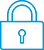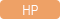cancel
Showing results for
Search instead for
Did you mean:It has been a while since anyone has replied. Simply ask a new question if you would like to start the discussion again.New member
1 0 0 0
Message 1 of 4
543
Flag Post

# Calculating the square of a negative number.HP Recommended
HP 300s+ Scientific Calculator

The HP 300s + Scientific Calculator incorrectly calculates the square of the negative number. After squaring the negative number, the result is negative. Mathematical nonsense.
Where am I making a mistake?

Tags (3)
3 REPLIES 3Level 8
747 744 121 187
Message 2 of 4
Flag PostHP Recommended

I would beleive your problem is that the - is being applied in a lower priority then ^2

So your input is being interpreted as -(5)^2 = -(25)

You can see that this actually working by typing -5 =, then do x^2 to get Ans^2. Evaluating that does return 25 like you'd expect.

TW

Although I work for the HP calculator group as a head developer of the HP Prime, the views and opinions I post here are my own.Level 8
622 609 117 207
Message 3 of 4
Flag PostHP Recommended

In case you are wondering whether only HP does this, please note that ALL modern calculators that let you enter -5² as -5² on a command line return -25 as the answer. I have a bunch of Casio and TI and Sharp calculators, and they all return -25, for the reason given by Tim above.

In fact, I'd go so far as to say that any calculator that returns positive 25 is buggy and not to be trusted, since it does not follow the standard order of operations (powers before negations).

Disclaimer: I don't work for HP. I'm just a calculator fanatic.

-Joe-Level 6
252 251 42 77
Message 4 of 4
Flag PostHP Recommended

Hello,

I understand the OP's frustration here. I have been struggeling with the same issue for a long time and have had to just accept this "modern" mathematic notation.

First let me abound in Joe's direction. The way the 300s does it is the standard way and is "correct" within the current math notation standards.

Here is how I rationalized it for myself.

Numbers can be positive or negative. In the past, the (-) key (or equivalent such as a normal - in front of the number that is not a negation operator) would be concidered as part of the number and indicate it's sign.

However, this is NOT how current math systems work anymore. They now concider the - as a prefix unary opeartor (ie an operation that operate on a single object and that is placed in front of said object). (² is a unary postfix opeartor. an operator that operates on a single object and placed after said object).

In lots of ways, this is way more powerfull than the old - as the operator can not apply to any type of mathematic object, including matrices, symbolic object, stuff in parenthesis...

However, there is a side effect in that priority of oeperators now affects the (-). and it's priority is lower than that of ² (no clue why honnestly).

The net effect of this is what you are seeing here. -2²=-4 and not 4! You can see it yourself using the leading math package: https://www.wolframalpha.com/input/?i=-2%C2%B2

Now, in lots of waysn the choise of operator priority is arbitrary, and I honneslty do not know why this is the order that was chosen by the math comunity. It definitely feel strange in this case, but, as I said, this is the standard.

Cyrille

I am an HP Employee
† The opinions expressed above are the personal opinions of the authors, not of HP. By using this site, you accept the Terms of Use and Rules of Participation